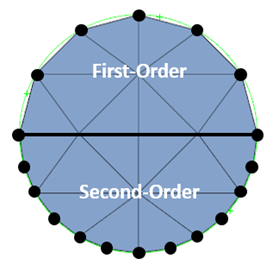Search

# First-Order vs. Second-Order Elements In FEA

Updated: Jul 22

Many of us are familiar with the terms first- and second-order as it relates to finite elements, but that doesn’t necessarily mean we all know how and when to use them. Today, we will be discussing a few key points regarding element order and making a case for why it matters to us as CAE analysts.

## What Are First- and Second-Order Elements?

Before we begin, it is important to remember exactly what a finite element model represents: a discretized depiction of a continuous system. Because this representation is comprised of discrete points (integration points) at which calculations are performed, values must be interpolated between them. To do this, the Finite Element Method (FEM) employs shape functions, which are mathematical relationships describing the behavior of a given element type. As with many things in Finite Element Analysis (FEA), these shape functions can assume either a linear (first-order) or non-linear (second-order) form. So, what exactly does that mean?Firstly, on the most fundamental level, it describes whether your elements contain only corner nodes (first-order) or if they contain corner nodes and mid-side nodes (second-order). More technically, though, it describes the behavior of the polynomial functions:

Linear (First-Order): P(x) = a*x + b

Quadratic (Second-Order): P(x) = a*x^2 + b*x + c

In layman’s terms, this just means that second-order elements are able to have curved edges whereas the edges of first-order elements must remain straight. As you can imagine, this means that quadratic elements are able to deform more realistically and can also more accurately capture geometric curvature (with fewer elements).## When Should I Use First- and Second-Order Elements?

As a general rule of thumb, it is better to use second-order elements over first-order – they deform more realistically than linear elements and can also better capture intricate geometric detail. However, this is a simplistic answer and neglects to consider the many reasons one might prefer to use first-order elements.

First-Order Elements

• Computationally Efficient

• Improved Contact Convergence

Second-Order Elements

• More Accurate Deformation

• Better Geometric Representation

• Computationally Expensive

## Why Does it Matter?

There are two key reasons why second-order elements are preferred over first: they are more accurate, and they can be more efficient depending on your meshing strategy. This point can be nicely illustrated using a simple handbook example:

Let us consider a bending problem in which a cantilever beam with a radius of 10 mm and a length of 100 mm is fixed on one side and loaded with 10,000 N on the opposing end. What should our value of maximum deflection be?

### Hand Calculation

First, we must calculate the area moment of inertia for our beam with a circular cross-section.

I = (π * Radius^4) / 4 = (3.14 * 10^4) / 4 = 7853.98 mm^4

Now that we know the area moment of inertia, we can use the following equation to calculate the maximum deflection in the beam:Deflection = (Load * Length^3) / (3 * Young’s Modulus * Moment of Inertia)

Deflection = (10000 * 100^3) / (3 * 200,000 * 7853.98) = 2.122 mm

### FEA Results

Next, let’s build a finite element representation of the problem above. In order to highlight the dangers of using first-order tets, sensitivity studies will be performed with tetrahedral elements only:

· Mesh Sizes: 1.0 mm, 2.0 mm, 4.0 mm

· Element Types: C3D4 (First-Order), C3D10 (Second-Order)

When using first-order elements (C3D4), the deflection is underpredicted, sometimes severely (11% in the example below). This occurs because first-order tetrahedral elements are too stiff; the constant displacement distribution in the element does not allow for accurate deformation. This phenomenon becomes more pronounced as element size increases and linear assumptions must be made over a larger area. As mesh size decreases, though, this issue can become negligible, as indicated by the 1.0 mm mesh results.## Final Thoughts

• Use second-order elements whenever possible.

• Don’t use first-order tetrahedral elements – they are too stiff (unless highly-refined, which is inefficient).

• First-order hexahedral elements provide an excellent blend of efficiency and accuracy.

As the example above illustrates, second-order elements provide more reliable results than their first-order counterparts. As such, it is recommended to use quadratic elements whenever possible. Of course, this may result in unbearable solve times, so engineers must balance accuracy and efficiency. Oftentimes, analysts will achieve this by blending high-quality, second-order mesh in areas of particular interest with coarsened, first-order mesh in areas of less concern. Using first-order hexahedral elements is another good way to marry accuracy and runtime efficiency.

If you need more help with any aspect of FEA, don't hesitate to get in touch with the team at Fidelis! This is our passion, and we're ready and waiting to help you with your next simulation project.

4,114 views

See All